基于奇异谱分析去噪和加权系数动态修正的风电功率实时组合预测模型Real Time Combination Forecasting Model of Wind Power Based on Singular Spectrum Denoising Analysis and Dynamic Correction of Weighting Coefficients

• 全文下载: PDF(1671KB)    PP.31-40   DOI: 10.12677/SG.2019.92004
• 下载量: 142  浏览量: 281

It is the essence of wind power forecasting to accurately grasp the variation law of wind power. However, most of the forecasting methods ignore the influence of noise on the wind power change law. Based on this, this paper proposes a real-time combination forecasting model of wind power based on singular spectrum denoising analysis and dynamic correction of weighting coefficients. In this model, the wind power time subsequence is decomposed by singular spectrum analysis, and the finite subsequence is obtained. Then, the number of the power spectral density wave peak of the wind power time subsequence is used as the distribution basis of the number of the recon-structed subsequence, and the noise elimination sequence is obtained and the residual amount is filtered as the noise. Finally, the self return is used. The weighted average dynamic correction combination model of sliding average, continuation method and least squares support vector ma-chine is used to predict the noise elimination sequence. The example analysis shows that this method can effectively improve the prediction accuracy and show good universality.

1. 引言

2. 奇异谱分析

1) 嵌入。设长度为N的一维时间序列 ${F}_{N}=\left({f}_{1},{f}_{2},\cdot \cdot \cdot ,{f}_{N}\right)$ ，给定正整数L为滑动窗口长度， $1 ，建立轨迹矩阵，可以表示为：

$X=\left[\begin{array}{l}{f}_{1}\text{}{f}_{2}\text{}\cdot \cdot \cdot \text{}{f}_{K}\\ {f}_{2}\text{}{f}_{3}\text{}\cdot \cdot \cdot \text{}{f}_{K+1}\\ \text{}⋮\text{}⋮\text{}⋮\text{}⋮\\ {f}_{L}\text{}{f}_{L+1}\text{}\cdot \cdot \cdot \text{}{f}_{N}\end{array}\right]$ (1)

2) 奇异谱分解。对轨迹矩阵X进行奇异谱分解，得到：

$X=\underset{i=1}{\overset{d}{\sum }}{\lambda }_{i}{U}_{i}{V}_{i}^{T}$ (2)

3) 分组。通常认为前r (r < d)个较大奇异值反映有用信号成分，后d - r个较小的奇异值被认为是噪声成分。这样，分组的操作就是确定合适的r值，从而实现信噪分离。

4) 对角平均化。实现矩阵转化为一维时间序列  的功能。重构序列可通过下式(3)获得，原序列由重构部分叠加如下式(4)计算得到：

${y}_{r{c}_{k}}=\left\{\begin{array}{ll}\frac{1}{k}\underset{m=1}{\overset{k}{\sum }}{y}_{m,k-m+1}^{\ast }\hfill & 1\le k<{L}^{\ast }\hfill \\ \frac{1}{{L}^{\ast }}\underset{m=1}{\overset{{L}^{*}}{\sum }}{y}_{m,k-m+1}^{\ast }\hfill & {L}^{\ast }\le k\le {K}^{\ast }\hfill \\ \frac{1}{N-k+1}\underset{m=k-{K}^{*}+1}{\overset{N-{K}^{*}+1}{\sum }}{y}_{m,k-m+1}^{\ast }\hfill & {K}^{\ast } (3)

${y}_{r}=\underset{k=1}{\overset{L}{\sum }}{y}_{r{c}_{k}}$ (4)

SSA在计算过程中有两个参数需要输入，即L和r，对重构序列进行分析确定 $L=N/2$  ，此时重构误差最小；原始信号的功率谱密度的主频谱个数n，选取 $r\text{=}2n$  。SSA能够对时间序列进行分解，将最可预报的分量集中分布到若干个具有重要意义的分量中进行重建，将噪声信号筛选出，从而实现信噪分离。

3. 加权系数动态修正组合模型

$\stackrel{^}{{P}_{t}}=\frac{{p}_{1t}+{p}_{2t}+\cdots +{p}_{nt}}{n}$ (5)

$\stackrel{^}{{P}_{t}}={c}^{1}{P}_{t}^{1}+\cdots +{c}^{n}{P}_{t}^{n}+{c}_{t}$ (6)

$Q=\mathrm{min}\underset{j=1}{\overset{d}{\sum }}{\left({T}_{jt}-\stackrel{^}{{P}_{jt}}\right)}^{2}$ (7)

$Q=\mathrm{min}\underset{j=1}{\overset{d}{\sum }}{\left({T}_{jt}-{c}_{t}^{1}{P}_{jt}^{1}-\cdots -{c}_{t}^{n}{P}_{jt}^{n}-{c}_{t}\right)}^{2}$ (8)

ct为第t时刻的预测组合权值，求取Q对ct的极值点，下一时刻，更新历史数据，重新计算权值，实现权值的动态实时修正，保持组合效果最佳状态，将计算得到的权值代回公式(7)，得到最终的风电出力预测结果。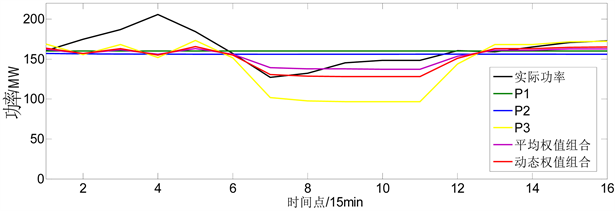Figure 1. Curve: forecasting resultsTable 1. Relative error of forecasting results

4. 基于奇异谱分析去噪和加权系数动态修正的风电功率实时组合预测模型

1) 原始风电功率时间序列F经过奇异谱分析的嵌入、分解、分组和对角平均化最终得到重构后的风电功率时间序列Y；

2) 对重构后的时间序列Y分别建立单一预测模型，各单一预测模型输出结果是 ${P}_{t}^{1},\cdots ,{P}_{t}^{n}$

3) 根据 $Q=\mathrm{min}\underset{j=1}{\overset{d}{\sum }}{\left({T}_{jt}-\stackrel{^}{{P}_{jt}}\right)}^{2}$ 建立第t预测时刻下组合权值的求解方程，其中 $\stackrel{^}{{P}_{jt}}={c}^{1}{P}_{jt}^{1}+\cdots +{c}^{n}{P}_{jt}^{n}+{c}_{t}$ ，风电实际出力 ${T}_{t}=\left\{{T}_{jt},j=1,\cdots ,d\right\}$ ，并求解出权值系数 ${c}_{t}^{1},\cdots ,{c}_{t}^{n},{c}_{t}$

4) 将步骤(3)求得的第t预测时刻下的权值系数引入公式(6)，便可计算获得未来第t预测时刻下的组合预测结果；

5) 下一时刻重新启动奇异谱分解与组合预测模型，更新训练样本数据，重复步骤(1)至步骤(4)，对权值进行动态修正，完成下一时刻的预测。

5. 算例分析

5.1. 数据来源Table 2. Installed capacity of wind farm

5.2. 评价指标

1) 日平均预测计划曲线准确率r1

${r}_{1i}=\left[1-\sqrt{\frac{1}{16}\underset{k=1}{\overset{16}{\sum }}{\left(\frac{{P}_{Mi}^{k}-{P}_{pi}^{k}}{Cap}\right)}^{2}}\right]×100%$ (9)

${r}_{1}=\frac{1}{96}\underset{i=1}{\overset{96}{\sum }}{r}_{1i}$ (10)

2) 全天预测结果均方根误差r2

${r}_{2}=\sqrt{\frac{1}{96×16}\underset{i=1}{\overset{96}{\sum }}\underset{k=1}{\overset{16}{\sum }}{\left(\frac{{P}_{Mi}^{k}-{P}_{Pi}^{k}}{Cap}\right)}^{2}}×100%$ (11)

3) 全天预测结果平均绝对误差r3

${r}_{3}=\frac{1}{96×16}\underset{i=1}{\overset{96}{\sum }}\underset{k=1}{\overset{16}{\sum }}|\frac{{P}_{Mi}^{k}-{P}_{Pi}^{k}}{Cap}|×100%$ (12)

r1i为第i次实时预测的准确率； ${P}_{Mi}^{k}$ 为第i次实时预测中第k个时刻的实际功率； ${P}_{Pi}^{k}$ 为第i次实时预测中第k个时刻的预测功率；Cap为风电场装机容量。

5.3. 实验结果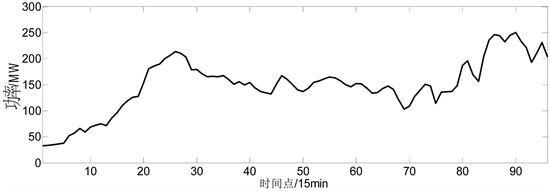Figure 2. Curve: original wind power output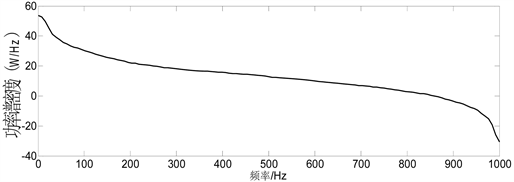Figure 3. Curve: power spectral density of wind power sequence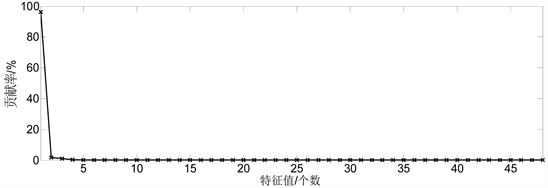Figure 4. Curve: eigenvalue contribution rate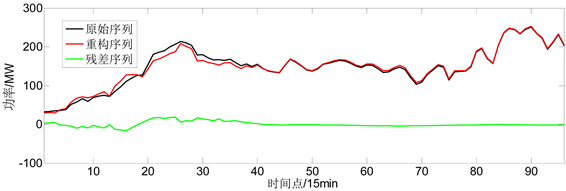Figure 5. Curve: diagram of original sequence, reconfiguration sequence and residual sequence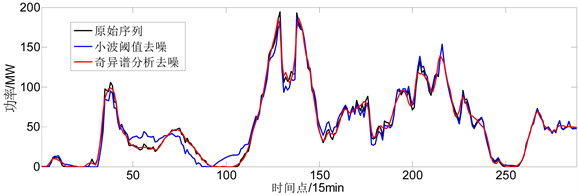Figure 6. Curve: schematic diagram of original sequence, wavelet threshold denoising and singular spectrum denoising analysisTable 3. Forecasting error results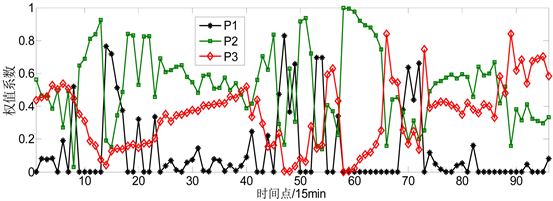Figure 7. Curve: combination coefficientTable 4. Forecasting error results of C wind farmTable 5. Forecasting error results of A wind farmTable 6. Forecasting error results of B wind farm

6. 结论

  刘波, 贺志佳, 金昊. 风力发电现状与发展趋势[J]. 东北电力大学学报, 2016, 36(2): 7-13.  杨茂, 刘红柳, 季本明. 基于混沌理论的风电功率超短期多步预测的误差分析[J]. 电力系统保护与控制, 2017, 45(4): 50-55.  叶林, 路朋, 滕景竹, 等. 考虑风电功率爬坡的功率预测–校正模型[J/OL]. 电力系统自动化: 1-8. http://kns.cnki.net/kcms/detail/32.1180.TP.20190201.1508.016.html, 2019-02-01.  匡洪海, 王建辉, 张瀚超, 等. 一种新型的风电功率爬坡段识别方法[J/OL]. 电网技术. https://doi.org/10.13335/j.1000-3673.pst.2018.1749, 2019-01-25.  杨茂, 陈新鑫, 张强, 等. 基于支持向量机的短期风速预测研究综述[J]. 东北电力大学学报, 2017, 37(4): 1-7.  杨茂, 黄宾阳, 江博, 等. 基于卡尔曼滤波和支持向量机的风电功率实时预测研究[J]. 东北电力大学学报, 2017, 37(2): 45-51.  肖迁, 李文华, 李志刚, 等. 基于改进的小波–BP神经网络的风速和风电功率预测[J]. 电力系统保护与控制, 2014, 42(15): 80-86.  叶瑞丽, 郭志忠, 刘瑞叶, 等. 基于小波包分解和改进Elman神经网络的风电场风速和风电功率预测[J]. 电工技术学报, 2017, 32(21): 103-111.  杨茂, 陈郁林. 基于EMD分解和集对分析的风电功率实时预测[J]. 电工技术学报, 2016, 31(21): 86-93.  Haque, A.U., Nehrir, M.H. and Mandal, P. (2014) A Hybrid Intelligent Model for Deterministic and Quantile Regression Approach for Probabilistic Wind Power Forecasting. Power System, 29, 1663-1672. https://doi.org/10.1109/TPWRS.2014.2299801  陈宁, 沙倩, 汤奕, 等. 基于交叉熵理论的风电功率组合预测方法[J]. 中国电机工程学报, 2012, 32(4): 29-34.  杨茂, 贾云彭, 钱为, 等. 基于动态权重的风电功率组合预测方法研究[J]. 东北电力大学学报, 2013, 33(1/2): 131-136.  Mahmoudvand, R. and Zokaei, M. (2012) On the Singular Values of the Hankel Matrix with Application in Singular Spectrym Analysis. Chilean Journal of Statistics, 3, 43-56.  征文, 程礼, 李应红. 利用奇异值分解的信号降噪方法[J]. 振动. 测试与诊断, 2011, 31(4): 459-463 + 534-535.